Q-Chem 5.0 User’s Manual

# A.6 Delocalized Internal Coordinates

We do not give further details of the optimization algorithms available in Q-Chem for imposing constraints in Cartesian coordinates, as it is far simpler and easier to do this directly in delocalized internal coordinates.

At first sight it does not seem particularly straightforward to impose any constraints at all in delocalized internals, given that each coordinate is potentially a linear combination of all possible primitives. However, this is deceptive, and in fact all standard constraints can be imposed by a relatively simple Schmidt orthogonalization procedure. In this instance consider a unit vector with unit component corresponding to the primitive internal (stretch, bend or torsion) that one wishes to keep constant. This vector is then projected on to the full set,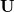, of active delocalized coordinates, normalized, and then all, for example, delocalized internals are Schmidt orthogonalized in turn to this normalized, projected constraint vector. The last coordinate taken in the active space should drop out (since it will be linearly dependent on the other vectors and the constraint vector) leaving (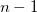) active vectors and one constraint vector.

In more detail, the procedure is as follows (taken directly from Ref. Baker:1996). The initial (usually unit) constraint vector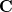is projected on to the setof delocalized internal coordinates according to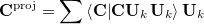(A.27)

where the summation is over allactive coordinates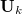. The projected vector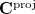is then normalized and an (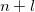) dimensional vector space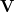is formed, comprising the normalized, projected constraint vector together with all active delocalized coordinates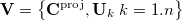(A.28)

This set of vectors is Schmidt orthogonalized according to the standard procedure,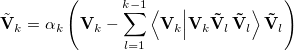(A.29)

where the first vector taken,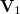, is. The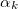in Eq. (A.29) is a normalization factor. As noted above, the last vector taken,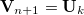, will drop out, leaving a fully orthonormal set of () active vectors and one constraint vector.

After the Schmidt orthogonalization the constraint vector will contain all the weight in the active space of the primitive to be fixed, which will have a zero component in all of the other () vectors. The fixed primitive has thus been isolated entirely in the constraint vector which can now be removed from the active subspace for the geometry optimization step.

Extension of the above procedure to multiple constraints is straightforward. In addition to constraints on individual primitives, it is also possible to impose combinatorial constraints. For example, if, instead of a unit vector, one started the constraint procedure with a vector in which two components were set to unity, then this would impose a constraint in which the sum of the two relevant primitives were always constant. In theory any desired linear combination of any primitives could be constrained.

Note further that imposed constraints are not confined to those primitive internals generated from the initial atomic connectivity. If we wish to constrain a distance, angle or torsion between atoms that are not formally connected, then all we need to do is add that particular coordinate to our primitive set. It can then be isolated and constrained in exactly the same way as a formal connectivity constraint.

Everything discussed thus far regarding the imposition of constraints in delocalized internal coordinates has involved isolating each constraint in one vector which is then eliminated from the optimization space. This is very similar in effect to a Z-matrix optimization, in which constraints are imposed by elimination. This, of course, can only be done if the desired constraint is satisfied in the starting geometry. We have already seen that the Lagrange multiplier algorithm, used to impose distance, angle and torsion constraints in Cartesian coordinates, can be used even when the constraint is not satisfied initially. The Lagrange multiplier method can also be used with delocalized internals, and its implementation with internal coordinates brings several simplifications and advantages.

In Cartesians, as already noted, standard internal constraints (bond distances, angles and torsions) are somewhat complicated non-linear functions of the,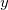andcoordinates of the atoms involved. A torsion, for example, which involves four atoms, is a function of twelve different coordinates. In internals, on the other hand, each constraint is a coordinate in its own right and is therefore a simple linear function of just one coordinate (itself).

If we denote a general internal coordinate by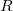, then the constraint function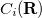is a function of one coordinate,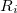, and it and its derivatives can be written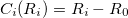(A.30)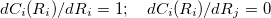(A.31)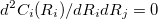(A.32)

where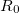is the desired value of the constrained coordinate, andis its current value. From Eq. (A.31) we see that the constraint normals,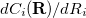, are simply unit vectors and the Lagrangian Hessian matrix, Eq. (A.21), can be obtained from the normal Hessian matrix by addingcolumns (androws) of, again, unit vectors.

A further advantage, in addition to the considerable simplification, is the handling of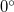and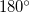dihedral angle constraints. In Cartesian coordinates it is not possible to formally constrain bond angles and torsions to exactlyorbecause the corresponding constraint normal is a zero vector. Similar difficulties do not arise in internal coordinates, at least for torsions, because the constraint normals are unit vectors regardless of the value of the constraint; thusanddihedral angle constraints can be imposed just as easily as any other value.bond angles still cause difficulties, but near-linear arrangements of atoms require special treatment even in unconstrained optimizations; a typical solution involves replacing a nearbond angle by two special linear co-planar and perpendicular bends , and modifying the torsions where necessary. A linear arrangement can be enforced by constraining the co-planar and perpendicular bends.

One other advantage over Cartesians is that in internals the constraint coordinate can be eliminated once the constraint is satisfied to the desired accuracy (the default tolerance is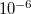in atomic units: Bohrs and radians). This is not possible in Cartesians due to the functional form of the constraint. In Cartesians, therefore, the Lagrange multiplier algorithm must be used throughout the entire optimization, whereas in delocalized internal coordinates it need only be used until all desired constraints are satisfied; as constraints become satisfied they can simply be eliminated from the optimization space and once all constraint coordinates have been eliminated standard algorithms can be used in the space of the remaining unconstrained coordinates. Normally, unless the starting geometry is particularly poor in this regard, constraints are satisfied fairly early on in the optimization (and at more or less the same time for multiple constraints), and Lagrange multipliers only need to be used in the first half-dozen or so cycles of a constrained optimization in internal coordinates.